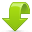# Query of Queries with maxrows bug

I found a bug today in CFMX, CF5 executes the code as you would expect.

Essentially if you do a query of query with maxrows, and then do another q of q on that result, the resulting q of q is using the wrong query and returns the wrong number of rows.

```<!--- make a query --->
<cfset q = QueryNew("a,b")>
<cfset r = QueryAddRow(q)>
<cfset QuerySetCell(q, "a", "a1", r)>
<cfset QuerySetCell(q, "b", "b2", r)>
<cfset r = QueryAddRow(q)>
<cfset QuerySetCell(q, "a", "a2", r)>
<cfset QuerySetCell(q, "b", "b3", r)>
<cfset r = QueryAddRow(q)>
<cfset QuerySetCell(q, "a", "a3", r)>
<cfset QuerySetCell(q, "b", "b1", r)>

<!--- dump it, 3 rows --->
<cfdump var="#q#">

<!--- select max rows of 2, 2 rows --->
<cfquery dbtype="query" name="result" maxrows="2">
SELECT * FROM q
ORDER BY a DESC
</cfquery>

<cfdump var="#result#">

<!--- select everything from the 2 row query returns 3 rows? --->
<cfquery dbtype="query" name="result2">
SELECT * FROM result
ORDER BY b DESC
</cfquery>

<cfdump var="#result2#" label="this should only have 2 rows, but it has 3">
```

I reported this to Macromedia, and they verified it as a bug.

#### Like this? Follow me ↯

This entry was:Download FuseGuard WAF for ColdFusion